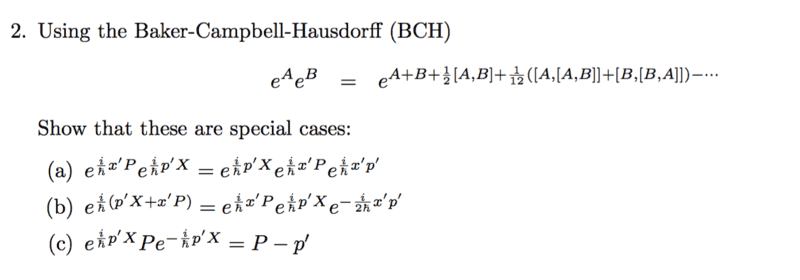# Special Cases of the BCH Identity

• WWCY
In summary, the problem is to find the momentum of a particle in a system of particles following the equation of motion

## Homework Statement

Hi all, I'm having trouble working on the following problem. Any assistance will be greatly appreciated.Here, the capital letters stand for Position and Momentum operators while the ##x', p'## stand for eigenvalues.

## The Attempt at a Solution

a) and b)

It seems that a) and b) can be written in the form of
$$e^{\frac{i}{\hbar}x'P}e^{\frac{i}{\hbar}p'X}$$
which I can then express in the form of the BCH identity
$$e^{\frac{i}{\hbar}x'P}e^{\frac{i}{\hbar}p'X} = e^{\frac{i}{\hbar}x'P + \frac{i}{\hbar}p'X + \frac{i}{2\hbar}x'p' + 0 + O'}$$
where I denote the other terms by ##O'##. If ##O' = 0##, I obtain b), if ##O' = \frac{i}{2\hbar}x'p' ##, I get a). Is this what the question was getting at? It does seem too convenient.

c)

Thanks in advance for any help!

WWCY said:

## Homework Statement

Hi all, I'm having trouble working on the following problem. Any assistance will be greatly appreciated.

View attachment 230761

Here, the capital letters stand for Position and Momentum operators while the ##x', p'## stand for eigenvalues.

## The Attempt at a Solution

a) and b)

It seems that a) and b) can be written in the form of
$$e^{\frac{i}{\hbar}x'P}e^{\frac{i}{\hbar}p'X}$$
which I can then express in the form of the BCH identity
$$e^{\frac{i}{\hbar}x'P}e^{\frac{i}{\hbar}p'X} = e^{\frac{i}{\hbar}x'P + \frac{i}{\hbar}p'X + \frac{i}{2\hbar}x'p' + 0 + O'}$$
where I denote the other terms by ##O'##. If ##O' = 0##, I obtain b), if ##O' = \frac{i}{2\hbar}x'p' ##, I get a). Is this what the question was getting at? It does seem too convenient.
It is not the best terminology to say that you express something "in the form of the BCH identity". People don't usually put it this way. What you have to do is to prove in each case that the left hand side is equal to the rhs, and the BCH identity can help you demonstrate this. So you should start from the expression on one side and end up on the expression on the other side, using any identity or algebraic manipulation you can do.

Focus on (a). Start from the left side and show that you get to the right side. This is almost what you did, but you did not complete your proof. You wrote

$$e^{\frac{i}{\hbar}x'P}e^{\frac{i}{\hbar}p'X} = e^{\frac{i}{\hbar}x'P + \frac{i}{\hbar}p'X + \frac{i}{2\hbar}x'p' + 0 + O'}$$

Now you must determine ##O'##. You cannot assume or choose the form it has. It has a unique expression and you must determine it. Once you will have shown what ##O'## is in this expression, you will have proven (a). Then we can discuss (b).

Hi @nrqed , I have actually managed to work out the problem, many thanks for your pointers!